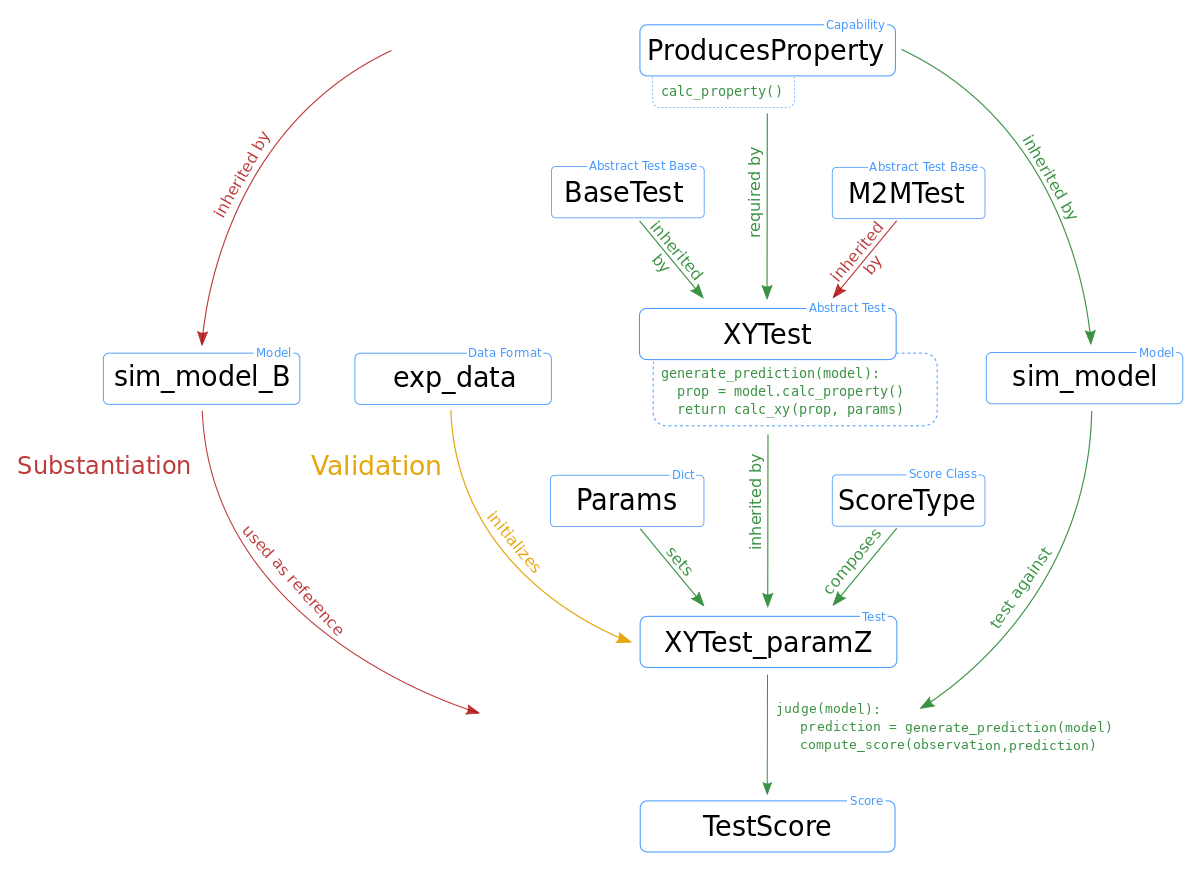# INM-6 / NetworkUnit

A SciUnit library for validation testing of neural network models.
Python

## Latest commit

Latest commit 181cc8a Dec 3, 2019

## Files

Type Name Latest commit message Commit time
Failed to load latest commit information.examplesfiguresnetworkunitACKNOWLEDGEMENTSAUTHORS.txtLICENSE.txtMANIFEST.inREADME.rstenvironment.ymlrequirements.txtsetup.py

# NetworkUnit

A SciUnit library for validation testing of spiking networks.## Installation

`pip install networkunit`

## Concept

The NetworkUnit module builds upon the formalized validation scheme of the SciUnit package, which enables the validation of models against experimental data (or other models) via tests. A test is matched to the model by capabilities and quantitatively evaluated by a score. The following figure illustrates a typical test design within NetworkUnit. The blue boxes indicate the components of the implementation of the validation test, i.e., classes, class instances, data sets, and parameters. The relation between the boxes are indicated by annotated arrows.The basic functionality is shown by green arrows. The difference in the test design for comparing against experimental data (validation) and another simulation (substantiation) is indicated by yellow and red arrows, respectively. The relevant functionality of some components for the computation of test score is indicated by pseudo-code. The capability class `ProducesProperty` contains the function `calc_property()`. The test `XYTest` has a function `generate_prediction()` which makes use of this capability, inherited by the model class, to generate a model prediction. The initialized test instance `XYTest_paramZ` makes use of its `judge()` function to evaluate this model prediction and compute the score `TestScore`. The `XYTest` can inherit from multiple abstract test classes (`BaseTest`), which is for example used with the `M2MTest` to add the functionality of evaluating multiple model classes. To make the test executable it has to be linked to a ScoreType and all free parameters need to be set (by a `Params` dict) to ensure a reproducible result.Showcase examples on how to use NetworkUnit can be found in this repository and interactive reveal.js slides are accessible via the launch-binder button at the top.

## Overview of tests

Class name Parent class Prediction measure
two_sample_test - -
correlation_test two_sample_test -
correlation_dist_test correlation_test correlation coefficients
correlation_matrix_test correlation_test correlation coefficient matrix
generalized_correlation_matrix_test correlation_matrix_test matrix of derived cross-correlation measures
eigenvalue_test correlation_test eigenvalues of the correlation coefficient matrix
covariance_test two_sample_test covariances
firing_rate_test two_sample_test firing rates
isi_variation_test two_sample_test inter-spike-intervals, their CV, or LV
graph_centrality_helperclass sciunit.Test graph centrality measures of given adjacency matrix

Inheritance order in case of multiple inheritance for derived test classes:

`class new_test(sciunit.TestM2M, graph_centrality_helperclass, <base_test_class>)`

## Overview of scores

Class name Test name Comparison measure
students_t Student't test sample mean
ks_distance Kolmogorov-Smirnov test sample distribution
kl_divergence Kullback-Leibler divergence sample entropy
mwu_statistic Mann-Whitney U test rank sum
levene_score Levene's test sample variance
effect_size Effect size standardized mean
best_effect_size Bayesian estimation effect size standardized mean

## Overview of model classes

Model name Capability Parent class Purpose